# If twice

If twice the age of the daughter is added to the age of the mother, the sum is 56. but if twice the age of the mother is added to the age of the daughter, the sum is 82. find the age of the mother and daughter.

d =  10
m =  36

### Step-by-step explanation:

2d+m=56
2m+d=82

2•d+m=56
2•m+d=82

2d+m = 56
d+2m = 82

d = 10
m = 36

Our linear equations calculator calculates it.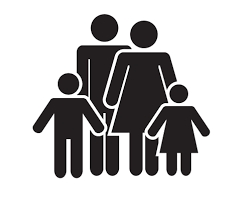Did you find an error or inaccuracy? Feel free to write us. Thank you!Tips to related online calculators
Do you have a system of equations and looking for calculator system of linear equations?
Do you want to convert time units like minutes to seconds?

## Related math problems and questions:

• Mother and daughter 2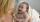The mother is 40 years older than her daughter. How old is the mother if her age is eight thirds age of daughter?
• Mother and daughter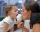Three years ago, the mother was three times older than the daughter. After nine years, she will be only twice old. How old is the mother (and daughter)?
• A motherA mother is three times older than her daughter. 9 years ago, mom was 6 times older than her daughter. How old are a mother and daughter now?
• Family and age ratiosThe age of father and son are in the ratio 10:3; the age of father and daughter are in the ratio 5:2. How old are father and son if the daughter is 20 years old? In what ratio is age sister and brother?
• Mother and daughterThe mother is four times older than her daughter. Five years ago, her daughter was seven times younger than her mother. How many years do they have now?
• Mother and daughterMother is 44 years old, her daughter 14. How many years ago was her mother four times older than her daughter?
• CarlaCarla is 5 years old, and Jim is 13 years younger than Peter. One year ago, Peter's age was twice the sum of Carla's and Jim's age. Find the present age of each one of them.
• Ages 2A man's age is 4 times his son's age. After 5 years he will be just twice his son's age, find their ages.
• Mother and daughter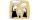The ratio of years mother and daughter is 5:2. After 7 years the ratio is 2: 1. How many years ago daughter was born?
• Reverse Pythagorean theoremGiven are lengths of the sides of the triangles. Decide which one is rectangular: Δ ABC: 77 dm, 85 dm, 36 dm ... Δ DEF: 55 dm, 82 dm, 61 dm ... Δ GHI: 24 mm, 25 mm, 7 mm ... Δ JKL: 32 dm, 51 dm, 82 dm ... Δ MNO: 51 dm, 45 dm,
• Sweets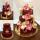3 chocolate and 7 cakes cost 85, - CZK. 2 chocolates and 6 cakes cost 86, - CZK. How much is 5 chocolates and 9 cakes? I wonder how to get the result, but only by logic without the use of a system of equations
• Time passing6 years ago, Marcela's mother was two times older than her and two times younger than her father. When Marcela is 36, she will be twice as young as her father. How old are Marcela, her father, and her mother now?
• Forth and back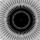Car drive from point A to point B at speed 78 km/h and back at 82 km/h. If went there and back at speed 81 km/h journey would take five minutes less. What is the distance between points A and B?
• Age of familyThe age of father and son is in the ratio 10: 3. The age of the father and daughter is in a ratio of 5: 2. How old are a father and a son if the daughter is 20?
• Chickens and rabbitsIn the yard were chickens and rabbits. Together they had 18 heads and 56 legs. How many chickens and how many rabbits were in the yard?
• An oilAn oil drum is cut in half. One half is used as a water trough. Use the dimensions; length 82cm, width 56cm to estimate the capacity of the water trough in liters.
• The sum 8The sum of two numbers is 21. If three times the smaller numbers is two less than twice the larger number, find the two numbers.